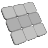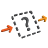Back to Adaptive Vision Library website

You are here: Start » Function Reference » Matrix

# Matrix

Select a function from the list below.

Icon Name Description LibraryAddMatrices Add two matrices of identical dimensions LiteCombineMatrices Combine two matrices horizontally LiteConcatenateMatrices Combine two matrices horizontally LiteCreateIdentityMatrix Create a square matrix with ones on the main diagonal, and zeros elsewhere. LiteCreateUniformMatrix Create a matrix with the specified dimensions and filled with an uniform element value. LiteInvertMatrix Find the inverse of a square matrix. LiteMultiplyMatrices Combine two matrices horizontally LiteMultiplyMatrixByReal Multiply all elements of a matrix by a value. Lite
SaveMatrix Saves serialized Matrix object as avdata file. LiteStackMatrices Combine two matrices vertically LiteSubtractMatrices Subtract two matrices of identical dimensions LiteTransposeMatrix Find the transpose of a matrix. Lite

Select Filter Equivalent below.

Icon Name Description LibraryAccessMatrix Return matrix dimensions and its elements row-by-row as a real array. LiteGetMatrixColumn Gets the values from a single column of a matrix. LiteGetMatrixElement Gets the value of a single element of a matrix. LiteGetMatrixRow Gets the values from a single row of a matrix. LiteMakeMatrix Create a matrix of specified dimensions with initial elements given row-by-row. LiteMatrixToRealArrayArray Converts a matrix to an array of its rows. LiteSetMatrixColumn Sets elements of a matrix column. LiteSetMatrixElement Sets a single element of a matrix. LiteSetMatrixRow Sets elements of a matrix row. LiteSkipEmptyMatrix Secures against domain errors caused by empty matrices. Lite